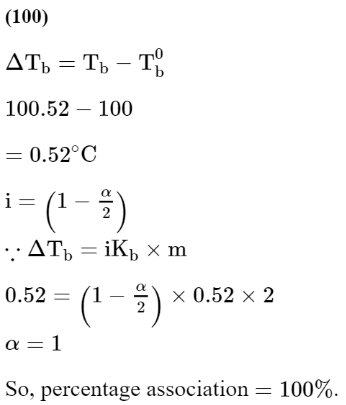# A solute a dimerizes in water.Question:

A solute a dimerizes in water. The boiling point of a 2 molar solution of A is $100.52^{\circ} \mathrm{C}$. The percentage association of $\mathrm{A}$ is _____________ . (Round off to the Nearest integer)

[Use : $\mathrm{K}_{\mathrm{b}}$ for water $=0.52 \mathrm{~K} \mathrm{~kg} \mathrm{~mol}^{-1}$ Boiling point of water $=100^{\circ} \mathrm{C}$ ]

Solution: